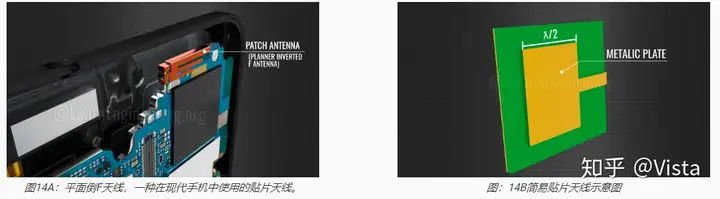Antenna

# How does an antenna work? What is the principle?

Antennas are widely used in telecommunications and we know that they have many applications. Antennas receive electromagnetic waves and convert them into electrical signals, or receive electrical signals and radiate them as electromagnetic waves. In this article, we’ll look at the science behind antennas.

The difference between fluctuating electromagnetic fields and radiated electromagnetic fields
We have electrical signals, so how do we convert them into electromagnetic waves? You can probably think of an easy answer. That is, using a closed conductor, and with the help of the principles of electromagnetic induction, you will be able to generate a fluctuating magnetic field and an electric field around it, as shown in Figure 1A. However, this fluctuating field around the source is of no use in transmitting the signal. Here the electromagnetic field doesn’t propagate; instead, it just fluctuates around the source. In an antenna, electromagnetic waves need to be separated from the source, and they should propagate (Figure: 1B). Before looking at how the antenna is made, let’s understand the physics behind wave separation.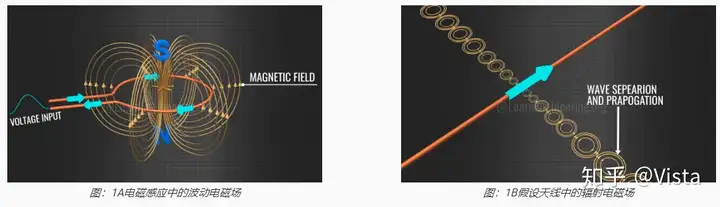The Physics Behind Oscillating Dipoles and Radiation
Consider a positive charge and a negative charge placed separately. Such arrangements are known as dipoles, and they obviously produce an electric field as shown in Figure 2A. Now, suppose these charges are oscillating, as shown in Figure 2B. At the midpoint of its path, the velocity will be maximum, and at the end of its path, the velocity will be zero. Due to this speed change, the charged particles undergo continuous acceleration and deceleration.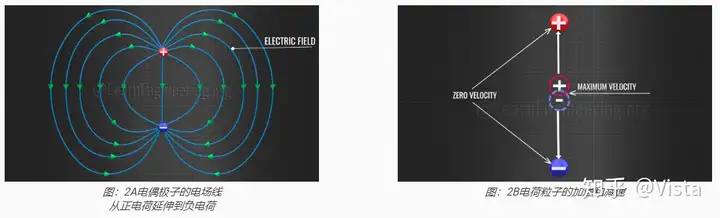1. Electric field lines at t = 0
The challenge now is to find out how the electric field changes due to this motion. Let’s focus on just one electric field line (Figure 3).Figure: 3 The electric field is shown at t = 0

2. Electric field lines at t = T/8
The wavefront formed at zero time period expanded and deformed as shown after one eighth time period (Fig. 4A). This is surprising; you might have expected a simple electric field, as shown in this location. Why does an electric field stretch and form such a field? As shown in Figure 4B, this is because accelerating or decelerating the charge will generate an electric field with a certain memory effect. Old electric fields are not easily adapted to new conditions. It takes us a while to understand the role of electric fields, the kinks that accelerate or decelerate charges.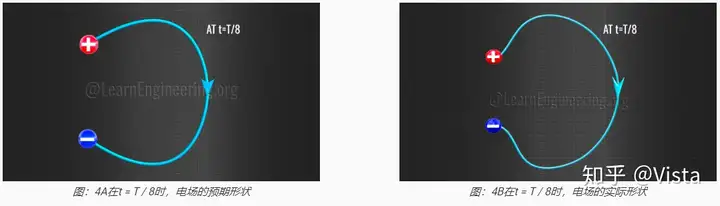3. Electric field lines at t = T/4
If we continue the analysis in the same way, we can see that for a quarter of the time period, the end points of the wave fronts meet at a single point (Figure 5).Figure: 5 At t = T/4, the ends of the electric fields meet at a single point, separation and propagation occur
Thereafter, separation and propagation of the wavefronts occurs. If you plot the electric field strength versus distance, you will see that the wave propagation is sinusoidal in nature (Figure 6). Interestingly, the resulting propagation wavelength is exactly twice the length of the dipole. We will come back to this later. Note that this changing electric field will automatically generate a changing magnetic field perpendicular to it. This is exactly what we need for our antenna. In short, if you can arrange to oscillate positive and negative charges, you can make an antenna.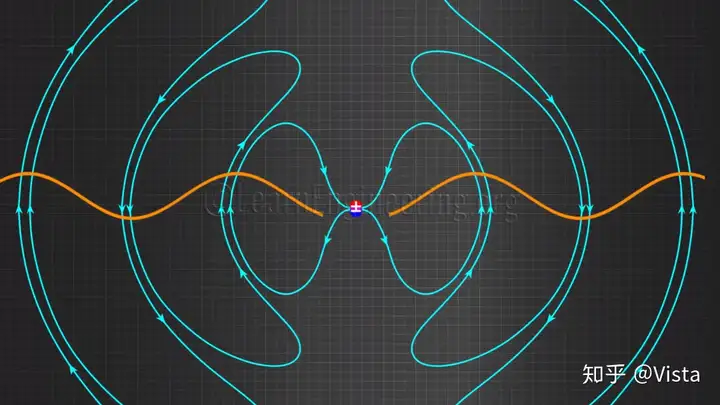Figure: Electromagnetic Radiation in 6 Dipoles

How does radiation in an antenna occur?
In fact, the generation of this oscillating charge is very easy. Take a conductive rod bent in the center and apply a voltage signal (7A) in the center. Let’s say this is your applied signal, a time-varying voltage signal. Consider the case of time zero. Due to the effect of the voltage, electrons will move from the right side of the dipole and accumulate on the left side. This means that the other end that loses electrons is automatically positively charged (7B). This arrangement creates the same effect as the previous case of dipole charges, positive and negative charges at the ends of the wire. As the voltage changes over time, the positive and negative charges will move back and forth.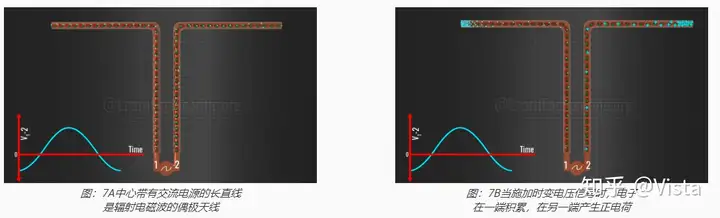A simple dipole antenna also produces the same phenomenon as in the previous section, and wave propagation occurs. Now we have seen how the antenna acts as a transmitter. The frequency of the transmitted signal will be the same as the frequency of the applied voltage signal. Since propagation travels at the speed of light, we can easily calculate the wavelength of propagation (Fig. 8). For perfect transmission, the length of the antenna should be half the wavelength.

ƒ Antenna = ƒ Input

C = ƒ antenna X ƛ antenna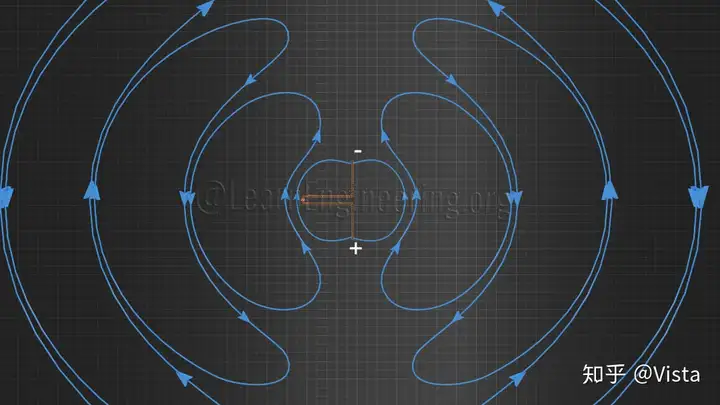Figure: 8 antennas radiate electromagnetic waves at the speed of light

How does the antenna receive the signal?
The operation of the antenna is reversible, and it can act as a receiver if a propagating electromagnetic field hits the antenna. Let’s look at this phenomenon in detail.

Use the same antenna again and apply an electric field. At this point, electrons will accumulate at one end of the rod. This is the same as an electric dipole. As the applied electric field changes, positive and negative charges build up at the other end. A varying charge build-up implies a varying voltage signal at the center of the antenna. As shown in Figure 9, when the antenna is used as a receiver, this voltage signal is the output. The frequency of the output voltage signal is the same as the frequency of the received electromagnetic wave. It is evident from the electric field configuration that for perfect reception the antenna should be half the size of the wavelength. In all these discussions, we have seen that the antenna is an open circuit.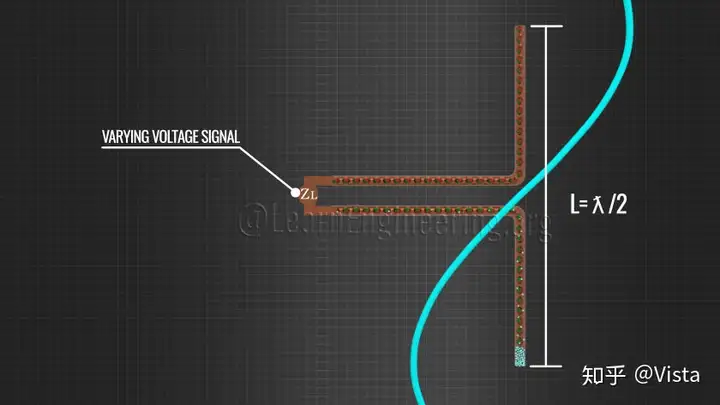Figure 9: An antenna can act as a receiver if a propagating electromagnetic field hits it
Antenna type

Now, let’s look at some practical antennas and how they work.

1. Yagi-Uda Antenna
In the past, dipole antennas were used for television reception. The colored bars act as dipoles and receive the signal as shown. The dipole is its main driving element. This antenna also requires a reflector and director to focus the signal on the dipole. The reflector element is always longer than the driven element and the director element is always shorter than the driven element. This complete structure is called the Yagi-Uda antenna (Fig. 10A). The Yagi antenna was invented by two Japanese scientists, Yagi Hidehisa and Uda Shintaro. It is a directional antenna, used for point-to-point communication. The driven element or dipole antenna converts the received signal into an electrical signal, which is transmitted to the TV unit via the coaxial cable (Figure 10B).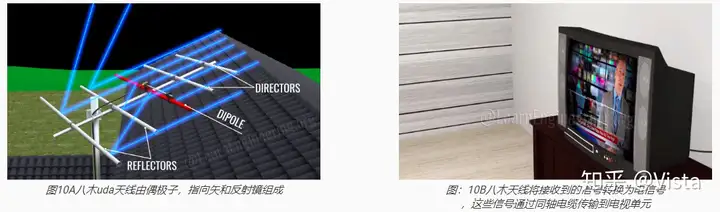2. Detailed Dish Antenna
Today, we have moved on to dish TV antennas. They consist of two main parts, a parabolic reflector and a low noise buck converter. The parabolic antenna receives the electromagnetic signal from the satellite and focuses it on the LNBF, as shown in Figure 11. The shape of the parabola is designed with particular precision.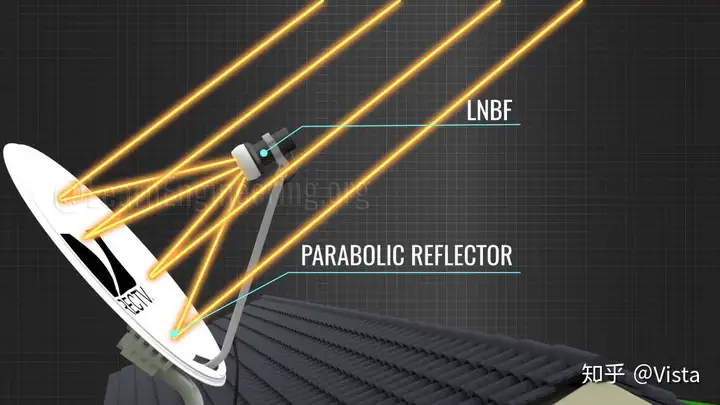Figure: 11 In a dish antenna, the incident signal is focused to the LNBF by a parabolic reflector
LNBF consists of feed horn, waveguide, PCB and probe (12A). The incoming signal is focused onto the probe through the feed horn and waveguide. As we saw in the simple dipole case, a voltage is induced at the probe. The voltage signal thus generated is fed to the PCB for signal processing such as filtering, conversion from high frequency to low frequency, and amplification. After signal processing, these electrical signals are transmitted to the TV unit via a coaxial cable (Figure 12B).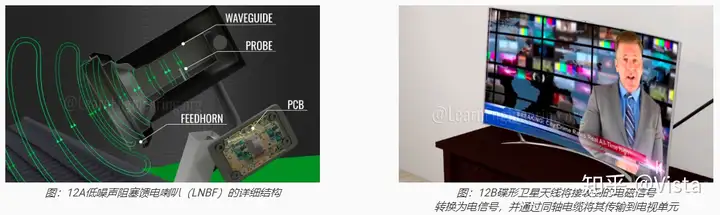If you open the LNB, you will most likely find 2 probes instead of one, with the second probe perpendicular to the first. The 2-probe arrangement means that the available spectrum can be used twice by sending horizontally or vertically polarized waves. As shown in Figure 13, one probe detects horizontally polarized signals and the other probe detects vertically polarized signals.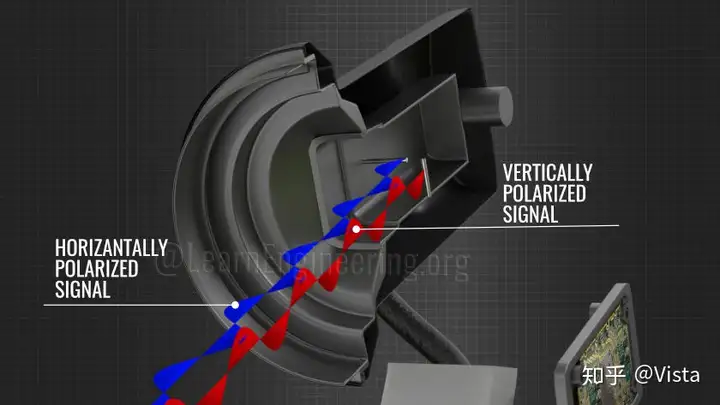Figure 13: Horizontal and vertical probes detect horizontally and vertically polarized signals, respectively.

3. Microstrip antenna or patch antenna
The phone in your hand uses a completely different type of antenna called a patch antenna (Figure 14A). These types of antennas are inexpensive and easy to manufacture on printed circuit boards. A patch antenna consists of a metal patch or strip placed on a ground plane with a piece of dielectric material in between. Here, metal patches are used as radiating elements. The length of the metal patch should be half the wavelength for proper transmission and reception (Figure 14B). Note that the description of the patch antenna we illustrate here is very basic.